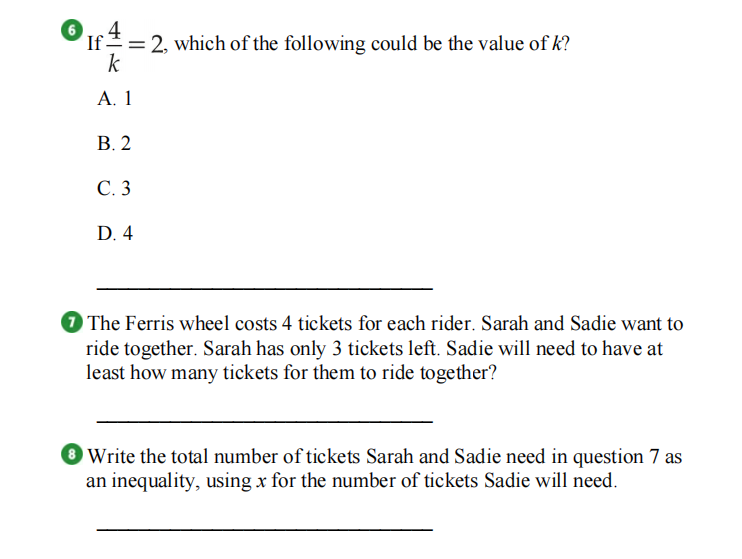### ¿Todavía tienes preguntas de matemáticas?

Pregunte a nuestros tutores expertos
Algebra
Pregunta6.If  $$\frac { 4 } { k } = 2$$ , which of the following could be the value of $$k$$ ? A. $$1$$ B. $$2$$ C. $$3$$ D. $$4$$

7. The Ferris wheel costs $$4$$ tickets for each rider. Sarah and Sadie want to ride together. Sarah has only $$3$$ tickets left. Sadie will need to have at least how many tickets for them to ride together?

8 .Write the total number of tickets Sarah and Sadie need in question $$7$$ as an inequality, using $$x$$ for the number of tickets Sadie will need.